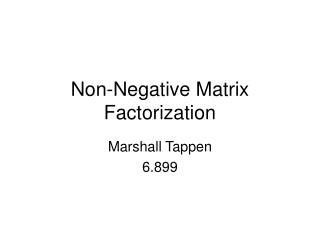DownloadDownload PresentationNon-Negative Matrix Factorization

# Non-Negative Matrix Factorization

Télécharger la présentation## Non-Negative Matrix Factorization

- - - - - - - - - - - - - - - - - - - - - - - - - - - E N D - - - - - - - - - - - - - - - - - - - - - - - - - - -
##### Presentation Transcript

1. Non-Negative Matrix Factorization Marshall Tappen 6.899

2. Problem Statement Given a set of images: • Create a set of basis images that can be linearly combined to create new images • Find the set of weights to reproduce every input image from the basis images • One set of weights for each input image

3. 3 ways to do this discussed • Vector Quantization • Principal Components Analysis • Non-negative Matrix Factorization • Each method optimizes a different aspect

4. Vector Quantization • The reconstructed image is the basis image that is closest to the input image.

5. What’s wrong with VQ? • Limited by the number of basis images • Not very useful for analysis

6. PCA Find a set of orthogonal basis images The reconstructed image is a linear combination of the basis images

7. What don’t we like about PCA? • PCA involves adding up some basis images then subtracting others • Basis images aren’t physically intuitive • Subtracting doesn’t make sense in context of some applications • How do you subtract a face? • What does subtraction mean in the context of document classification?

8. Non-negative Matrix Factorization • Like PCA, except the coefficients in the linear combination cannot be negative

9. NMF Basis Images • Only allowing adding of basis images makes intuitive sense • Has physical analogue in neurons • Forcing the reconstruction coefficients to be positive leads to nice basis images • To reconstruct images, all you can do is add in more basis images • This leads to basis images that represent parts

10. PCA Designed for producing optimal (in some sense) basis images Just because it’s optimal doesn’t mean it’s good for your application NMF Designed for producing coefficients with a specific property Forcing coefficients to behave induces “nice” basis images No SI unit for “nice” PCA vs NMF

11. The cool idea • By constraining the weights, we can control how the basis images wind up • In this case, constraining the weights leads to “parts-based” basis images

12. Objective function • Let the value of a pixel in an original input image be V. Let (WH)iµ be the reconstructed pixel. • If we consider V to be a noisy version of (WH)iµ , then the PDF of V is • Now we will maximize the log probability of this PDF over W and H, leaving the relevant objective function to be:

13. How do we derive the update rules? • This is in the NIPS paper. • (I’m going to change the error function to to match the NIPS paper) • Use gradient descent to find a local minimum • The gradient descent update rule is:

14. Deriving Update Rules • Gradient Descent Rule: • Set • The update rule becomes

15. What’s significant about this? • This is a multiplicative update instead of an additive update. • If the initial values of W and H are all non-negative, then the W and H can never become negative. • This lets us produce a non-negative factorization • (See NIPS Paper for full proof that this will converge)

16. How do we know that this will converge? • If F is the objective function, let be G be an auxiliary function • If G is an auxiliary function of F, then F is non-increasing under the update

17. Auxiliary Function

18. How do we know that this will converge? • Let the auxiliary function be • Then the update is • Which results in the update rule:

19. Main Contributions • Idea that representations which allow negative weights do not make sense in some applications • A simple system for producing basis images with non-negative weights • Points out that this leads to basis images that are based on parts • A larger point here is that by constraining the problem in new ways, we can induce nice properties

20. Mel’s Commentary • Most significant point: • “NMF’s non-negativity constraint is well-matched to our intuitive ideas about decomposition into parts” • Second: Basis images are better • Third: Simple System

21. Mel’s Caveats • Comparison of NMF to PCA is subjective • Basis images don’t necessarily correspond to parts as we think of them. • Subtraction may actually occur in the brain • Some neurons are “negative” versions of others

22. A Quick Review of Linear Algebra • Every vector can be expressed as the linear combination of basis vectors • Can think of images as big vectors (Raster scan image into vector) • This means we can express an image as the linear combination of a set of basis images

23. Where do those update rules come from??? • How this is a matrix problem X Basis Images (W) Reconstruction Weights (H) The basis pixels that will be combined into the reconstructed pixel Weights used to combine basis images Into reconstructed pixel